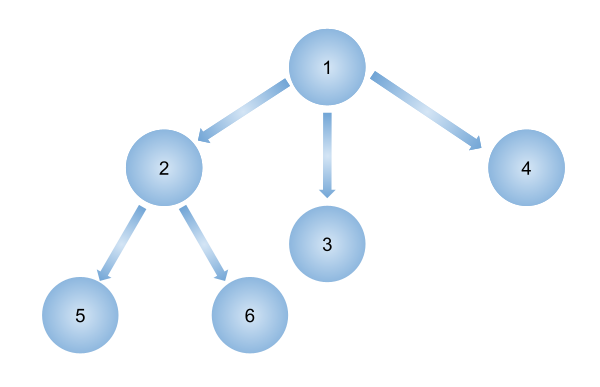# N-ary tree Level Order Traversal

Posted: 25 Mar, 2021
Difficulty: Moderate

## PROBLEM STATEMENT

#### You are given an N-ary tree where every node has at most ‘N’ child nodes. You need to find the level order traversal of this tree

##### Note:
``````Note that the level order traversal must not contain any null values, simply return the tree in level order.
``````
##### Input Format:
``````The first line contains an integer 'T' which denotes the number of test cases or queries to be run. Then the test cases are as follows.

The first line of each test case contains elements of the N-ary tree in the level order form. The line consists of values of nodes separated by a single space. In case a node is changed, we take -1. To get next node
The first not-null node(of the previous level) is treated as the parent of the first node of the current level. The second not-null node (of the previous level) is treated as the parent node for the next nodes of the current level and so on.

The input ends when all nodes at the last level are null(-1).
``````
##### Note :
``````The above format was just to provide clarity on how the input is formed for a given tree.
The sequence will be put together in a single line separated by a single space. Hence, for the below-depicted tree, the input will be given as:
1 2 3 4 -1 5 6 -1 -1 -1 -1 -1
``````##### Output Format:
``````For each test case, you need to print the level order traversal of the tree.

Print the output of each test case in a separate line.
``````
##### Note:
``````You don’t need to print anything; It has already been taken care of. Just implement the given function.
``````
##### Constraints:
``````1 <= T <= 10
1 <= K <= 10^4

Time limit: 1 sec
``````Approach 1

In this approach, we will simply apply BFS and store the current level nodes of the tree in a queue, and finally push them to the resultant vector.

Algorithm:

• Create a vector/list to store the 'RESULT' or the level order traversal of the tree.
• Create a base case:
• If NODE == NULL, where ‘NODE' is the current node
• Return ‘RESULT’
• Create a queue to keep the current level nodes and their children.
• Push the current node in the queue.
• Start traversing until the queue has a value:
• Create a vector to store elements of the current level
• Push children of current level nodes into the queue
• And one by one add current level only nodes in a vector
• After pushing all children of current level into queue AND pushing current level nodes(parent nodes) into vector
• Push that vector storing current level nodes in the 'RESULT' vector
• Return RESULT